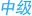返回首页客户留言联系我们

E-mail: 2669691914@qq.com

d=铜线径  G=铜比重  N=条数  K1=铜线绞入率  K2=芯线绞入率  C=绝缘芯线根数

D=绝缘外径  d=导体外径  G=绝缘比重  K2=芯线绞入率  C=绝缘芯线根数

D1=完成外径  D=上过程外径  G=绝缘比重

D=上过程外径  t=包带厚度  G=包带比重  Z=重叠率(1/4Lap = 1.25)

d=铜线径  N=条数  G=比重  Z=绞入率
6.编织用量：（Kg/Km）= d^2 * 0.7854 * T * N * G / cosθ
θ = atan( 2 * 3.1416 * ( D + d * 2 )) * 目数 / 25.4 / T
d=编织铜线径  T=锭数  N=每锭条数  G=铜比重

PVC-1.45；LDPE-0.92；HDPE-0.96；PEF（发泡）-0.65；FRPE-1.7；Teflon（FEP）2.2；Nylon-0.97；PP-0.97；PU-1.21电话：86-0316-5962058 手机：13833657342### 化工仪器网4